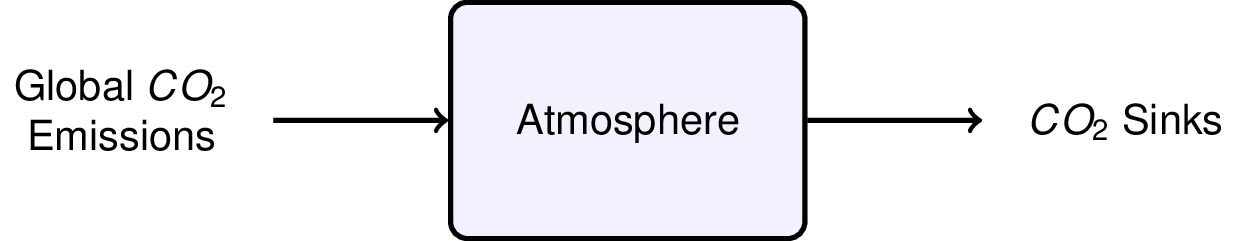This notebook contains course material from CBE20255 by Jeffrey Kantor (jeff at nd.edu); the content is available on Github. The text is released under the CC-BY-NC-ND-4.0 license, and code is released under the MIT license.

# Global CO2 Budget¶

## Summary¶

This Jupyter notebook provides an analysis of global CO2 emissions go by solving a mass balance.

## Problem Statement¶

The total global emissons of CO2 is estimated by the Netherlands Environmental Assessment Agency to be 34.5 billion metric tons from all sources, including fossil fuels, cement production, and land use changes. As measured by NOAA, in recent years the atmospheric concentration of CO2 is increasing at an annual rate of about 2.4 ppmv (parts per million by volume).Assuming these numbers are accurate and that the atmosphere is well mixed, what fraction of global CO2 emissions are being retained in the atmosphere?

## Solution¶

This problem requires us to perform a material balance for CO2 in the atmosphere. We'll perform the using a 10 step approach outlined in the textbook.

### Step 1. Draw a diagram.¶### Step 2. Define the system of interest.¶

The system of interest is the global atmosphere which we assume is well mixed and of uniform composition.

### Step 3. Choose components and define stream variables.¶

The chemical component to model is CO2. The stream variables are the mass flowrates of CO2</sub> into and out of the atmosphere.

### Step 4. Convert all units to consistent units of mass or moles.¶

This problem could be done on either a molar or mass basis. We'll arbitrarily choose to do this in mass units of kg/year. First we convert global emissions to kg/year.

In [ ]:
%matplotlib inline
from numpy import *

In [ ]:
mCO2_in = 34.5e9            # inflow, metric tonnes per year
mCO2_in = mCO2_in*1000      # inflow, kg per year
print("Global CO2 emissions = {:8.3g} kg/yr".format(mCO2_in))

Global CO2 emissions = 3.45e+13 kg/yr


The rate of accumulation is given as ppm by volume per year. For ideal gases, volume fraction is equivalent to mole fraction. We need to convert the mole fraction, which is the ratio of kg-moles of CO2 to kg-moles of air, to mass fraction which has units of kg of CO2 to kg of air per year.

In [ ]:
nCO2_accum = 2.4e-6                   # accumulation, kg-mol CO2/kg-mol air/yr

mwAir = 28.97                         # kg air/kg-mol air
mwCO2 = 44.01                         # kg CO2/kg-mol CO2

wCO2_accum = nCO2_accum*mwCO2/mwAir   # kg CO2/kg air/yr

print("Accumulation Rate of CO2 = {:8.3g} kg CO2/kg air".format(wCO2_accum))

Accumulation Rate of CO2 = 3.65e-06 kg CO2/kg air


### Step 5. Define a basis.¶

The basis for the calculation are flows and change in one year. No additional work is required.

### Step 6. Define system variables.¶

The system variable is the rate of accumulation of CO2 in kg CO2/year. We need to convert from change in concentration per year to change in total mass per year. The first step is to estimate the total mass of air.

In [ ]:
# Earth Radius in meters
R = 6371000       # m

# Earth Area in square meters
A = 4*pi*R**2     # m**2

# Mass of the atmosphere in kg
g = 9.81          # N/kg
P = 101325        # N/m**2
mAir = A*P/g      # kg

print("Estimated mass of the atmosphere = {:8.3g} kg".format(mAir))

Estimated mass of the atmosphere = 5.27e+18 kg


To get the rate of change of total CO2, multiply the total mass of by the rate of change of mass fraction of CO2.

In [ ]:
mCO2_accum = wCO2_accum*mAir     # kg CO2/year

print("Change in CO2 = {:8.3g} kg CO2/year".format(mCO2_accum))

Change in CO2 = 1.92e+13 kg CO2/year


### Step 7. List specifications¶

The inflow and rate of change of CO2 are specified, and calculated above.

### Step 8. Write material balance equations for each species.¶

$$\mbox{Accumulation} = \mbox{Inflow} - \mbox{Outflow} + \underbrace{\mbox{Generation}}_{=0} - \underbrace{\mbox{Consumption}}_{=0}$$

### Step 9. Solve material balance equations.¶

$$\mbox{Outflow} = \mbox{Inflow} - \mbox{Accumulation}$$
In [ ]:
mCO2_out = mCO2_in - mCO2_accum

print("Global CO2 outflow = {:8.3g} kg CO2/yr".format(mCO2_out))

Global CO2 outflow = 1.53e+13 kg CO2/yr


### Step 10. Check your work.¶

Fraction retained in the atmosphere

In [ ]:
fCO2 = mCO2_accum/mCO2_in
print("Fraction of CO2 retained in the atmosphere = {:<.2g} ".format(fCO2))

Fraction of CO2 retained in the atmosphere = 0.56

In [ ]: